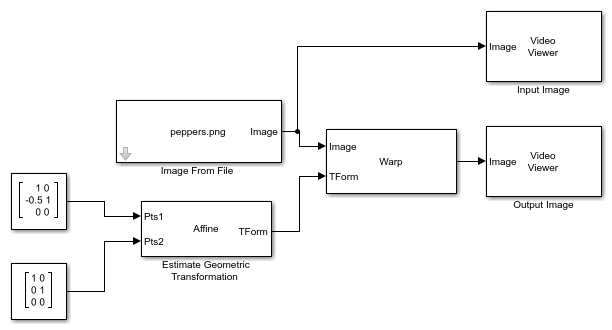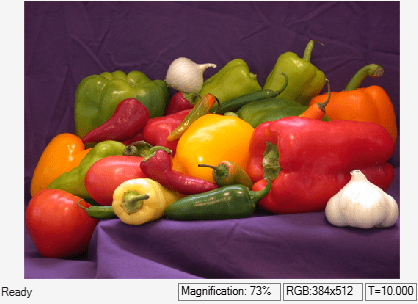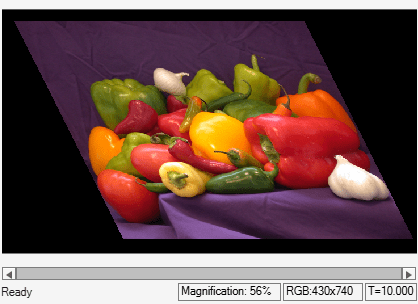# Apply Affine Transformation to Image

This example shows you how to perform a horizontal shear transformation of an image by calculating an affine transformation matrix.

### Example Model

```modelname = "ex_blkestimategeometrictransformation.slx"; open_system(modelname) ```This model reads an input image using an Image From File block with the File name parameter set to `peppers.png`.

To estimate the Affine transformation matrix, this model uses the Estimate Geometric Transformation block with default parameters.

To apply a horizontal shear transformation to the input image, the model uses a Warp block that accepts the input image at the Image input port and the estimated transformation matrix at the TForm input port. The block then horizontally shears the image by using these parameters:

• Transformation matrix source`Input port`.

• Interpolation method`Bilinear`

• Background fill value`0`

• Output image position source`Custom`

• Output image position vector [x y width height]`[-20 -20 740 430]`

### Simulate the Model

Run the model. The Video Viewer blocks display the input image and the transformed output image.

```sim(modelname); ```